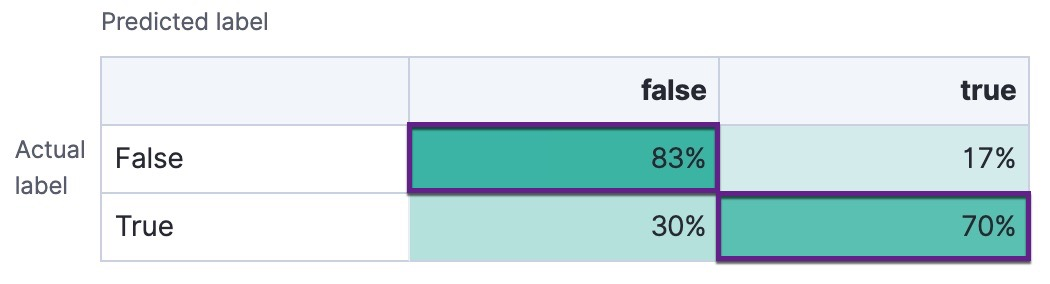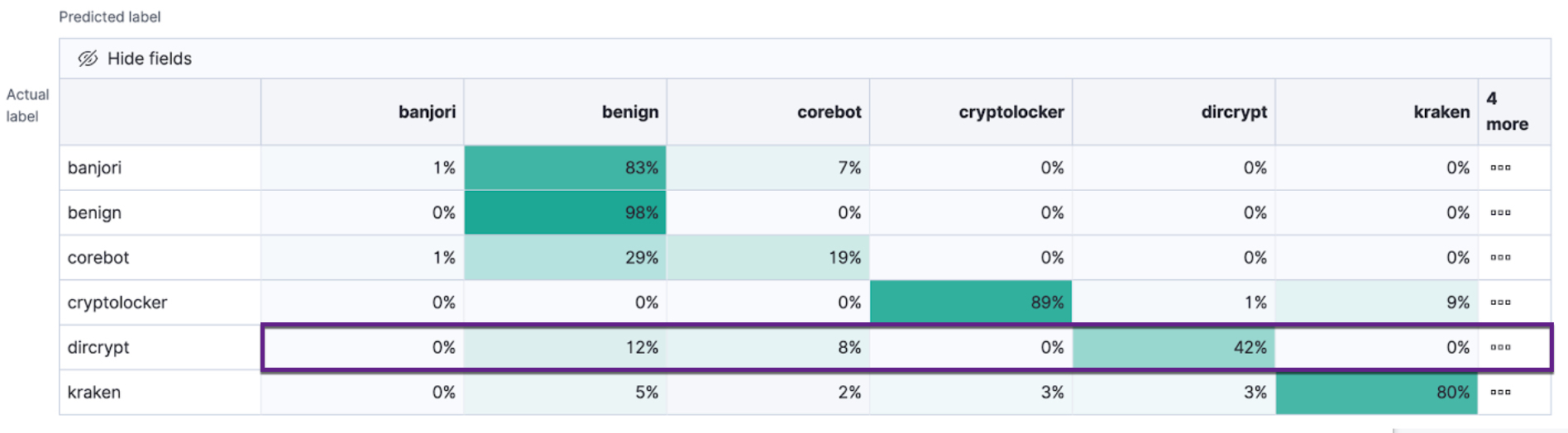Classificationedit

Classification is a machine learning process that enables you to predict the class or category of a data point in your data set. Typical examples of classification problems are predicting loan risk, classifying music, or detecting the potential for cancer in a DNA sequence. In the first case, for example, the data set might contain the investment history, employment status, debit status, and other financial details for loan applicants. Based on this data, you could use classification analysis to create a model that predicts whether it is safe or risky to lend money to applicants. In the second case, the data contains song details that enable you to classify music into genres like hip-hop, country, or classical, for example. Classification is for predicting discrete, categorical values, whereas regression analysis predicts continuous, numerical values.

When you create a classification job, you must specify which field contains the classes that you want to predict. This field is known as the dependent variable. It must contain no more than 30 classes. By default, all other supported fields are included in the analysis and are known as feature variables. The runtime and resources used by the job increase with the number of feature variables. Therefore, you can optionally include or exclude fields from the analysis. For more information about field selection, see the explain data frame analytics API.

Training the classification modeledit

Classification – just like regression – is a supervised machine learning process. When you create the data frame analytics job, you must provide a data set that contains the ground truth. That is to say, your data set must contain the dependent variable and the feature variables fields that are related to it. You can divide the data set into training and testing data by specifying a training_percent. By default when you use the create data frame analytics jobs API, 100% of the eligible documents in the data set are used for training. If you divide your data set, the job stratifies the data to ensure that both the training and testing data sets contains classes in proportions that are representative of the class proportions in the full data set.

When you are collecting a data set to train your model, ensure that it captures information for all of the classes. If some classes are poorly represented in the training data set (that is, you have very few data points per class), the model might be unaware of them. In general, complex decision boundaries between classes are harder to learn and require more data points per class in the training data.

When you create a data frame analytics job, the inference step of the process might fail if the model is too large to fit into JVM. For a workaround, refer to this GitHub issue.

Classification algorithmsedit

Classification analysis uses an ensemble algorithm that is a type of boosting called boosted tree regression model which combines multiple weak models into a composite one. It uses decision trees to learn to predict the probability that a data point belongs to a certain class. A sequence of decision trees are trained and every decision tree learns from the mistakes of the previous one. Every tree is an iteration of the last one, hence it improves the decision made by the previous tree.

Deploying the modeledit

The model that you created is stored as Elasticsearch documents in internal indices. In other words, the characteristics of your trained model are saved and ready to be deployed and used as functions. The inference feature enables you to use your model in a preprocessor of an ingest pipeline or in a pipeline aggregation of a search query to make predictions about your data.

Classification performanceedit

As a rule of thumb, a classification analysis with many classes takes more time to run than a binary classification process when there are only two classes. The relationship between the number of classes and the runtime is roughly linear.

The runtime also scales approximately linearly with the number of involved documents below 200,000 data points. Therefore, if you double the number of documents, then the runtime of the analysis doubles respectively.

To improve the performance of your classification analysis, consider using a smaller training_percent value when you create the job. That is to say, use a smaller percentage of your documents to train the model more quickly. It is a good strategy to make progress iteratively: use a smaller training percentage first, run the analysis, and evaluate the performance. Then, based on the results, decide if it is necessary to increase the training_percent value. If possible, prepare your input data such that it has less classes. You can also remove the fields that are not relevant from the analysis by specifying excludes patterns in the analyzed_fields object when configuring the data frame analytics job.

Interpreting classification resultsedit

The following sections help you understand and interpret the results of a classification analysis. To see example results, refer to Viewing classification results.

class_probabilityedit

The class_probability is a value between 0 and 1, which indicates how likely it is that a given data point belongs to a certain class. The higher the number, the higher the probability that the data point belongs to the named class. This information is stored in the top_classes array for each document in your destination index.

class_scoreedit

The class_score is a function of the class_probability and has a value that is greater than or equal to zero. It takes into consideration your objective (as defined in the class_assignment_objective job configuration option): accuracy or recall.

If your objective is to maximize accuracy, the scores are weighted to maximize the proportion of correct predictions in the training data set. For example, in the context of a binary confusion matrix with classes false and true, the predictions of interest are the cells where the actual and predicted labels are both true (also known as a true positive (TP)) or both false (also known as a true negative (TN)):If there is an imbalanced distribution of classes in your training data set, focusing on accuracy can decrease your model’s sensitivity to incorrect predictions in the classes that are under-represented in your data.

By default, classification analysis jobs accept a slight degradation of the overall accuracy in return for greater sensitivity to classes that are predicted incorrectly. That is to say, their objective is to maximize the minimum recall. For example, in the context of a multi-class confusion matrix, the predictions of interest are in each row:For each class, the recall is calculated as the number of correct predictions (where the actual label matches the predicted label) divided by the sum of all the other predicted labels in that row. This value is represented as a percentage in each cell of the confusion matrix. The class scores are then weighted to favor predictions that result in the highest recall values across the training data. This objective typically performs better than accuracy when you have highly imbalanced data.